• 傅里叶变换matlab FFT 函数解析 傅里叶变换网上有很多资料了，本博客主要讲实现《信号系统》P181页，不同周期的方波 的傅里叶级数： 与sinc形成包络： 其间隔ω0，如下图： 本博客用matlab 实现一下。 下面...
傅里叶变换 和 matlab FFT 函数解析
傅里叶变换网上有很多资料了，本博客主要讲实现《信号系统》P181页，不同周期的方波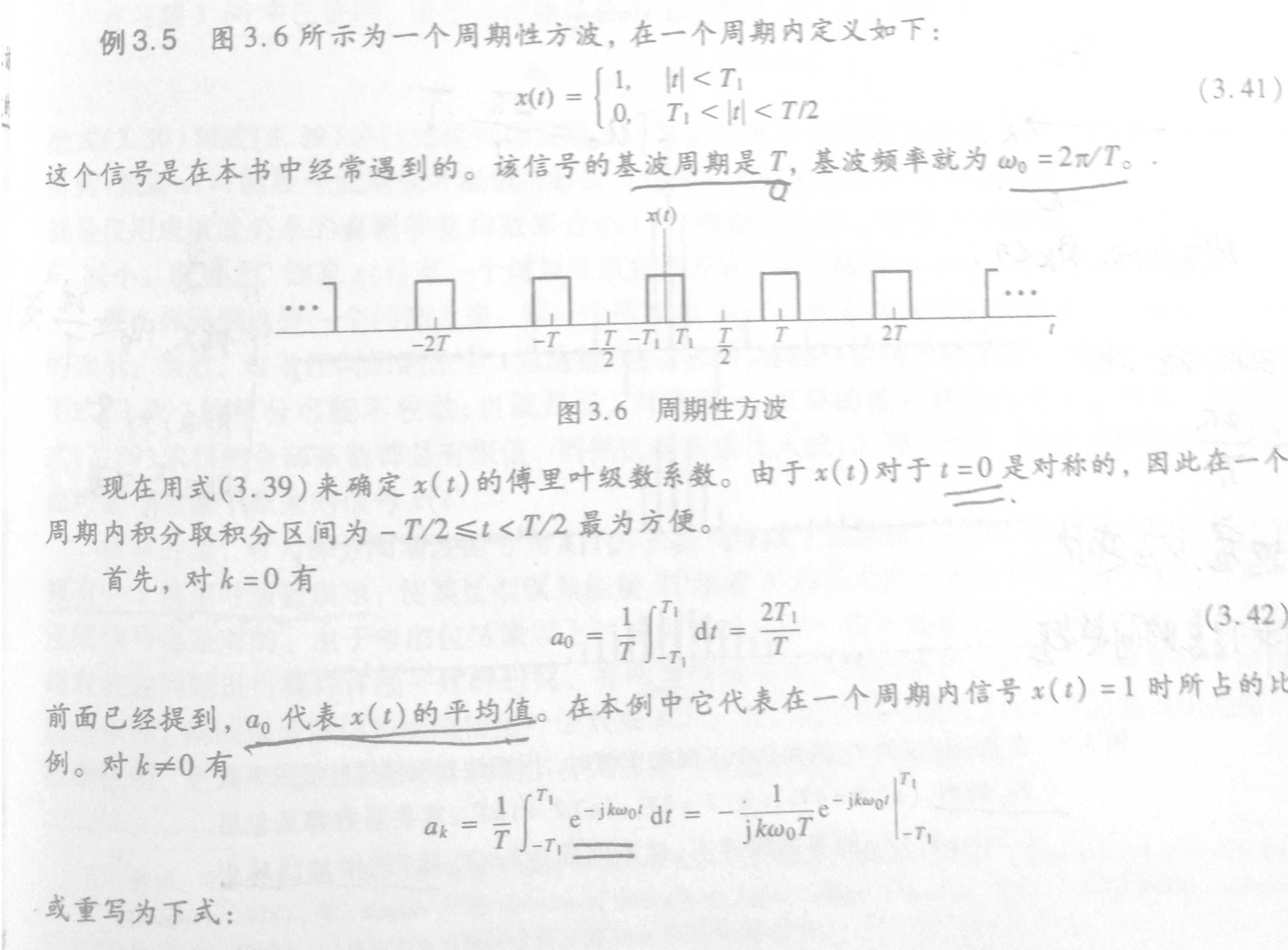的傅里叶级数：与sinc形成包络：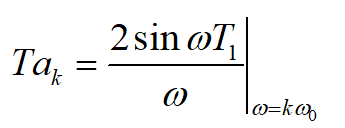其间隔ω0，如下图：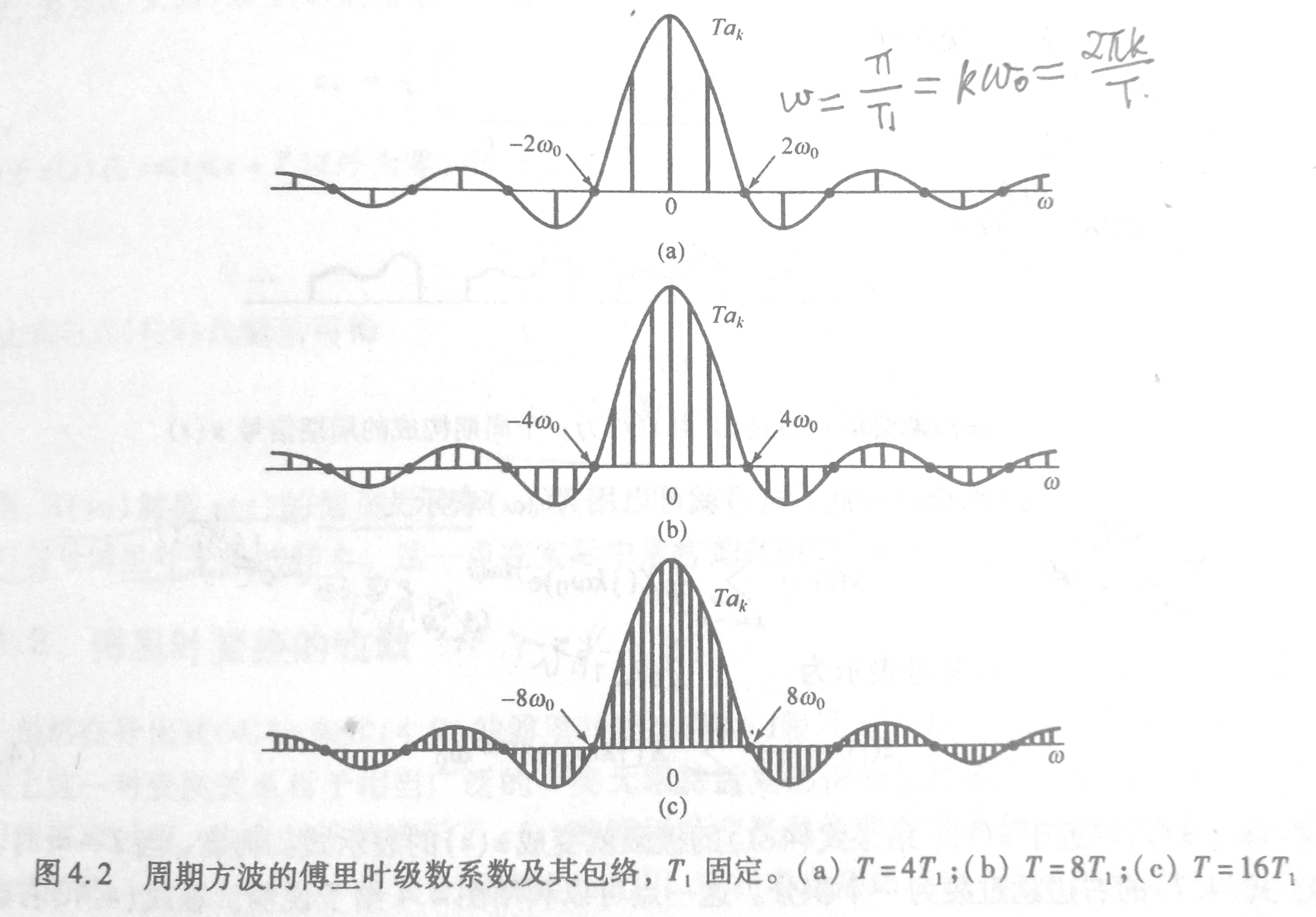本博客用matlab 实现一下。
下面解析matlab fft
matlab fft 函数用于离散快速傅里叶变换，输出一个与输入等长n的序列。
它按以下方式计算：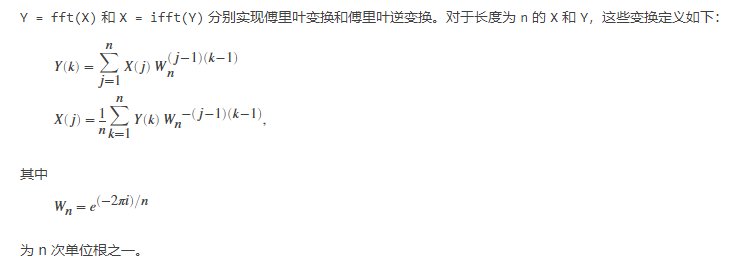有此公式也可以自行实现fft，例如实部：
clear
t0 = 0.1;% 采样间隙
tr = 32;
t = -tr:t0:tr;
n = length(t);
y = 3*cos(2*t);
mY = zeros(size(t));
w = linspace(-1/t0*pi,1/t0*pi,n);
for k = 1:n
for j = 1:n
mY(k) = mY(k) + y(j)*cos(-2*pi*(j-1)*(k-1)/n);
end
end
Y = fft(y);
plot(real(Y)-mY)

对比连续的傅里叶级数展开公式：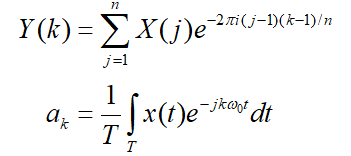设n是全部样本数，t0是时间间隔，则有以下转换方式：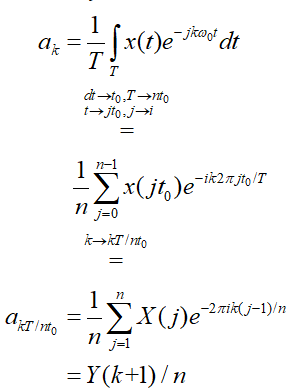那么傅里叶系数ak对应fft()函数结果有如下关系：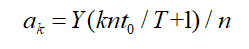这说明ak在fft()结果中间隔nt0/T，可以离散fft用来离散化连续傅里叶级数。
代码
基础设置
clear
t0 = 0.01;% 采样间隙
tr = 3200;
t = -tr:t0:tr;%样本时长，注意tr必须为T的整数倍，保证周期完整！
T1 = 0.1;%单周期方波时长
T = T1*16;%方波周期，改变T为4T1,8T1,16T1对应上面三个图
n = length(t); %离散化的点数，也对应fft结果的点数
y = zeros(size(t)); %信号
w = linspace(-1/t0*pi,1/t0*pi,n);% 频域范围对应2pi/t0
% ω 间隔2pi/(t0n),对应ak间隔2pi/(t0n)*nt0/T= ω0 与书上结论一致

产生占空比为2T1/T的方波：
for i = 1:n
if abs((rem(t(i),T))) < T1 || abs((rem(t(i),T)))>=T-T1
y(i) = 1;
end
end
figure()
plot(t,y);

调整T，画包络图
figure()
plot(w,2*sin(w*T1)./w) %sinc
hold on
Y = fft(y)/n;
k = fftshift(Y); %fftshift 将Y(0)移动到中心，Y(n/2:end)移动到负数频域
plot(w,T*real(k)) %频谱（包含ak，间隔nt0/T）

实验结果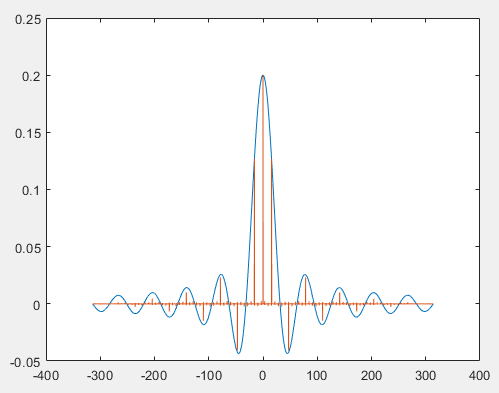T = 4T1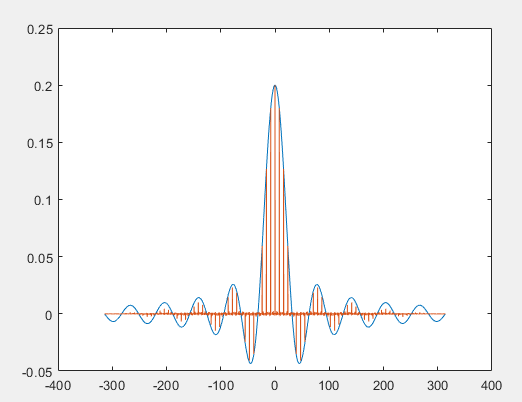T = 8T1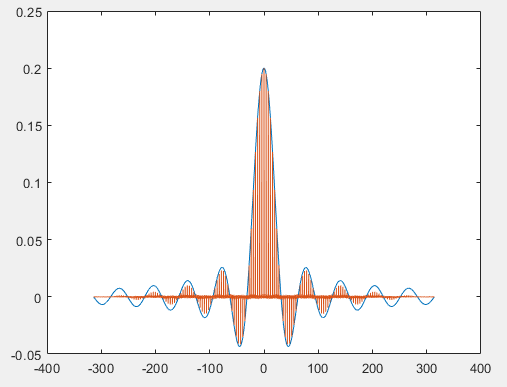T = 16T1
参考：

<<信号与系统>>
https://ww2.mathworks.cn/help/matlab/ref/fft.html


展开全文信号处理
• 傅里叶变换，缩写为FFT变换，是数字信号处理领域一种很重要的算法。要知道傅立叶变换算法的意义，首先要了解傅立叶原理的意义。傅立叶原理表明：任何连续测量的时序或信号，都可以表示为不同频率的正弦波信号的无限...
傅里叶变换，缩写为FFT变换，是数字信号处理领域一种很重要的算法。要知道傅立叶变换算法的意义，首先要了解傅立叶原理的意义。傅立叶原理表明：任何连续测量的时序或信号，都可以表示为不同频率的正弦波信号的无限叠加。而根据该原理创立的傅立叶变换算法利用直接测量到的原始信号，以累加方式来计算该信号中不同正弦波信号的频率、振幅和相位。和傅立叶变换算法对应的是反傅立叶变换算法。该反变换从本质上说也是一种累加处理，这样就可以将单独改变的正弦波信号转换成一个信号。因此，可以说，傅立叶变换将原来难以处理的时域信号转换成了易于分析的频域信号(信号的频谱)，可以利用一些工具对这些频域信号进行处理、加工。最后还可以利用傅立叶反变换将这些频域信号转换成时域信号。一个模拟信号，经过ADC采样之后，就变成了数字信号。 采样得到的数字信号，就可以做FFT变换了。N个采样点，经过FFT之后，就可以得到N个点的FFT结果。为了方便进行FFT运算，通常N取2的整数次方。假设采样频率为Fs，信号频率F，采样点数为N。那么FFT之后结果就是一个为N点的复数。每一个点就对应着一个频率点。这个点的模值，就是该频率值下的幅度特性。具体跟原始信号的幅度有什么关系呢？假设原始信号的峰值为A，那么FFT的结果的每个点(除了第一个点直流分量之外)的模值就是A的N/2倍。而第一个点就是直流分量，它的模值就是直流分量的N倍。而每个点的相位呢，就是在该频率下的信号的相位。第一个点表示直流分量(即0Hz)，而最后一个点N的再下一个点(实际上这个点是不存在的，这里是假设的第N+1个点，也可以看做是将第一个点分做两半分，另一半移到最后)则表示采样频率Fs，这中间被N-1个点平均分成N等份，每个点的频率依次增加。由此可见，FFT频谱图中所能达到的分辨率为为Fs/N，即某点n所表示的频率为：Fn=(n-1)*Fs/N。如果采样频率Fs为1024Hz，采样点数为1024点，则可以分辨到1Hz。假设FFT之后某点n用复数a+bi表示，那么这个复数的模就是An=sqrt(a*a+b*b)(某点处的幅度值An = A*(N/2))，相位就是Φ=atan2(b,a)。根据以上的结果，就可以计算出n点(n≠1，且n<=N/2)对应的信号的表达式为：2*An/N*cos(2*pi*Fn*t+Φ)。对于n=1点的信号，是直流分量，幅度即为A1/N。由于FFT结果的对称性，通常我们只使用前半部分的结果，即小于采样频率一半的结果。下面以上一篇文章的信号来做说明f0=10;fs=80;N=64;n=0:N-1;y=sin(2*pi*n*f0/fs);则matlab程序为f0=10;fs=80;N=64;n=0:N-1;y=sin(2*pi*n*f0/fs);y1=fft(y);z=abs(y1);plot(n,z);图形为：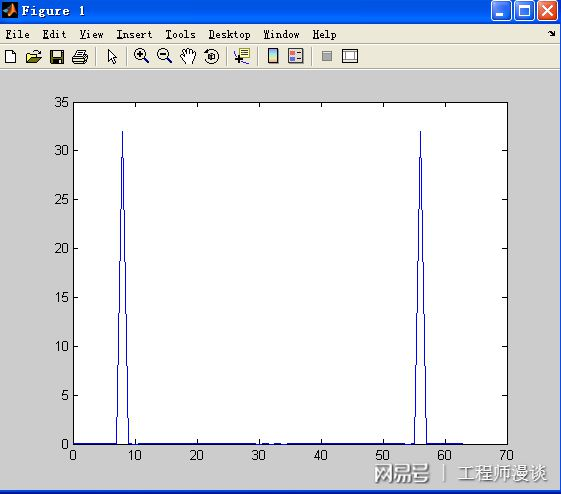由图形可知 在n = 8时有最大值32，可以验证上述是否成立，并且验证x[n]是否与x[t]有对应关系。因为An = A*(N/2)，所以A = 1因为频谱分辨率为fs/N = 1.25，所以fs = n*fs/N = 8*1.25 = 10，与原函数相同如果想在图形中直接显示原函数的频率需要将n轴归一化,如下图所示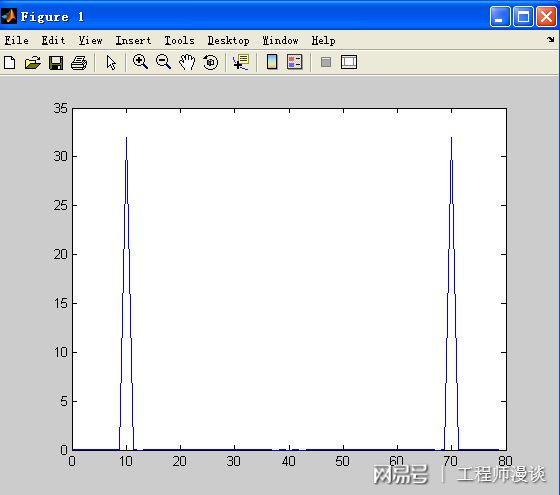以上就是正弦函数及其FFT变换的全部内容了，你学会了没有？
展开全文• 描述一种积分变换，它来源于函数傅里叶积分表示。积分 (1) 称为ƒ 的傅里叶积分。周期函数在一定条件下可以展成傅里叶级数，而在(－∞，∞)上定义的非周期函数ƒ，显然不能用三角级数来表示。但是J.-B.-J.傅里叶...
描述一种积分变换，它来源于函数的傅里叶积分表示。积分 (1) 称为ƒ 的傅里叶积分。周期函数在一定条件下可以展成傅里叶级数，而在(－∞，∞)上定义的非周期函数ƒ，显然不能用三角级数来表示。但是J.-B.-J.傅里叶建议把ƒ表示成所谓傅里叶积分的方法。傅里叶变换在物理学、电子类学科、数论、组合数学、信号处理、概率论、统计学、密码学、声学、光学、海洋学、结构动力学等领域都有着广泛的应用(例如在信号处理中，傅里叶变换的典型用途是将信号分解成幅值谱——显示与频率对应的幅值大小)。傅里叶变换(fft)matlab程序一Fs = 128; % 采样频率T = 1/Fs; % 采样时间L = 256; % 信号长度t = (0:L-1)*T; % 时间x = 5 + 7*cos(2*pi*15*t - 30*pi/180) + 3*cos(2*pi*40*t - 90*pi/180); %cos为底原始信号y = x + randn(size(t)); %添加噪声 figure; plot(t，y)title(‘加噪声的信号’)xlabel(‘时间(s)’)N = 2^nextpow2(L); %采样点数，采样点数越大，分辨的频率越精确，N》=L，超出的部分信号补为0Y = fft(y，N)/N*2; %除以N乘以2才是真实幅值，N越大，幅值精度越高f = Fs/N*(0:1：N-1); %频率A = abs(Y); %幅值P = angle(Y); %相值figure;subplot(211);plot(f(1:N/2)，A(1:N/2)); %函数fft返回值的数据结构具有对称性，因此我们只取前一半title(‘幅值频谱’);xlabel(‘频率(Hz)’);ylabel(‘幅值’);subplot(212);plot(f(1:N/2)，P(1:N/2));title(‘相位谱频’);xlabel(‘频率(Hz)’);ylabel(‘相位’);傅里叶变换(fft)matlab程序二tp=0:2048; % 时域数据点数N yt=sin(0.08*pi*tp).*exp(-tp/80); % 生成正弦衰减函数plot(tp，yt)， axis(［0，400，-1，1］)， % 绘正弦衰减曲线t=0:800/2048:800; % 频域点数Nff=0:1.25:1000;yf=fft(yt); % 快速傅立叶变换ya=abs(yf(1:801)); % 幅值yp=angle(yf(1:801))*180/pi; % 相位 yr=real(yf(1:801)); % 实部yi=imag(yf(1:801)); % 虚部figure subplot(2，2，1)plot(f，ya)，axis(［0，200，0，60］) % 绘制幅值曲线title(‘幅值曲线’)subplot(2，2，2)plot(f，yp)，axis(［0，200，-200，10］) % 绘制相位曲线title(‘相位曲线’)subplot(2，2，3)plot(f，yr)，axis(［0，200，-40，40］) % 绘制实部曲线title(‘实部曲线’)subplot(2，2，4)plot(f，yi)，axis(［0，200，-60，10］) % 绘制虚部曲线title(‘虚部曲线’)结果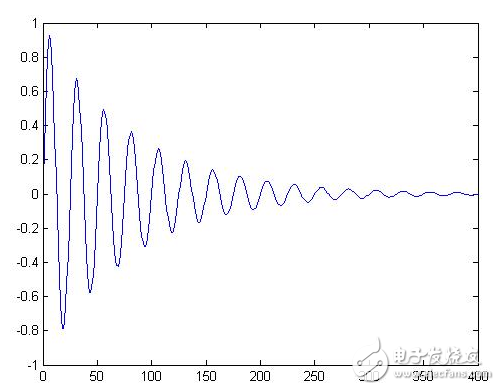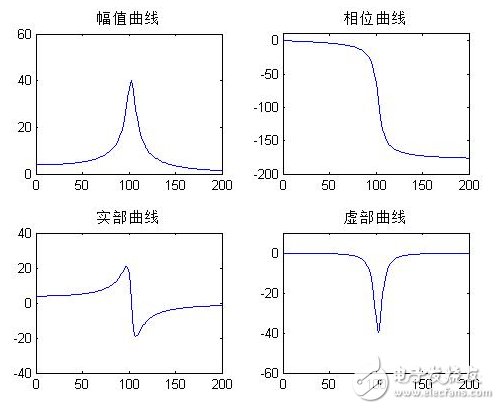傅里叶变换(fft)matlab程序三clear all %清除内存所有变量close all %关闭所有打开的图形窗口%% 执行FFT点数与原信号长度相等(100点)% 构建原信号N=100; % 信号长度(变量@@@@@@@)Fs=1; % 采样频率dt=1/Fs; % 采样间隔t=［0:N-1］*dt; % 时间序列xn=cos(2*pi*0.24*［0:99］)+cos(2*pi*0.26*［0:99］);xn=［xn，zeros(1，N-100)］; % 原始信号的值序列subplot(3，2，1) % 变量@@@@@@@plot(t，xn) % 绘出原始信号xlabel(‘时间/s’)，title(‘原始信号(向量长度为100)’) % 变量@@@@@@@% FFT分析NN=N; % 执行100点FFTXN=fft(xn，NN)/NN; % 共轭复数，具有对称性f0=1/(dt*NN); % 基频f=［0:ceil((NN-1)/2)］*f0; % 频率序列A=abs(XN); % 幅值序列subplot(3，2，2)，stem(f，2*A(1:ceil((NN-1)/2)+1))，xlabel(‘频率/Hz’) % 绘制频谱(变量@@@@@@@)axis(［0 0.5 0 1.2］) % 调整坐标范围title(‘执行点数等于信号长度(单边谱100执行点)’); % 变量@@@@@@@%% 执行FFT点数大于原信号长度% 构建原信号N=100; % 信号长度(变量@@@@@@@)Fs=1; % 采样频率dt=1/Fs; % 采样间隔t=［0:N-1］*dt; % 时间序列xn=cos(2*pi*0.24*［0:99］)+cos(2*pi*0.26*［0:99］);xn=［xn，zeros(1，N-100)］; % 原始信号的值序列subplot(3，2，3) % 变量@@@@@@@plot(t，xn) % 绘出原始信号xlabel(‘时间/s’)，title(‘原始信号(向量长度为100)’) % 变量@@@@@@@% FFT分析NN=120; % 执行120点FFT(变量@@@@@@@)XN=fft(xn，NN)/NN; % 共轭复数，具有对称性f0=1/(dt*NN); % 基频f=［0:ceil((NN-1)/2)］*f0; % 频率序列A=abs(XN); % 幅值序列subplot(3，2，4)，stem(f，2*A(1:ceil((NN-1)/2)+1))，xlabel(‘频率/Hz’) % 绘制频谱(变量@@@@@@@)axis(［0 0.5 0 1.2］) % 调整坐标范围title(‘执行点数大于信号长度(单边谱120执行点)’); % 变量@@@@@@@%% 执行FFT点数与原信号长度相等(120点)% 构建原信号N=120; % 信号长度(变量@@@@@@@)Fs=1; % 采样频率dt=1/Fs; % 采样间隔t=［0:N-1］*dt; % 时间序列xn=cos(2*pi*0.24*［0:99］)+cos(2*pi*0.26*［0:99］);xn=［xn，zeros(1，N-100)］; % 原始信号的值序列subplot(3，2，5) % 变量@@@@@@@plot(t，xn) % 绘出原始信号xlabel(‘时间/s’)，title(‘原始信号(向量长度为120)’) % 变量@@@@@@@% FFT分析NN=120; % 执行120点FFT(变量@@@@@@@)XN=fft(xn，NN)/NN; % 共轭复数，具有对称性f0=1/(dt*NN); % 基频f=［0:ceil((NN-1)/2)］*f0; % 频率序列A=abs(XN); % 幅值序列subplot(3，2，6)，stem(f，2*A(1:ceil((NN-1)/2)+1))，xlabel(‘频率/Hz’) % 绘制频谱(变量@@@@@@@)axis(［0 0.5 0 1.2］) % 调整坐标范围title(‘执行点数等于信号长度(单边谱120执行点)’); % 变量@@@@@@@结果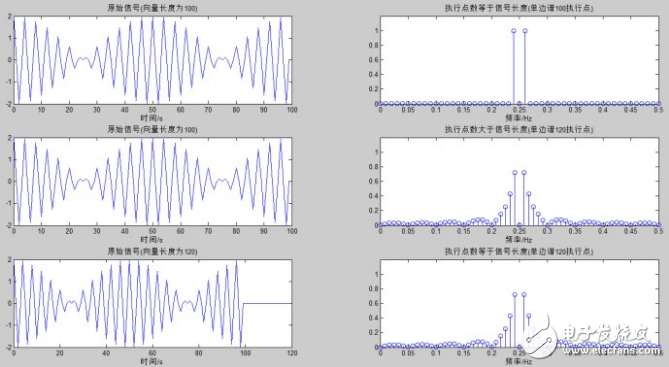打开APP阅读更多精彩内容点击阅读全文
展开全文• Matlab 已经有 fft 和 ifft 来快速计算离散傅立叶变换及其逆。 但是它们是二进制内置函数，因此它们的代码不可见，这对想要了解 FFT 工作原理的人没有帮助。 为了教学和学习的目的，我认为我们还应该有一对可读的...
• 傅里叶变换 通过傅里叶变换，可以将混合信号解析成叠加的不同频率信号的振幅 代码见博客最后方 算法实现 请编写 1024 点快速傅里叶变换的算法。自行生成一段混杂若干不同频率正弦的信号，测试所编写的快速...
傅里叶变换

通过傅里叶变换，可以将混合信号解析成叠加的不同频率信号的振幅

代码见博客最后方

算法实现

请编写 1024 点快速傅里叶变换的算法。自行生成一段混杂若干不同频率正弦的信号，测试所编写的快速傅里叶变换算法。

设计思路

首先生成一个由多个不同频率的正弦函数叠加的信号，这里我的频率都是小于1/2pi的，因此其中必有周期2pi
再在2pi周期里面等分取1024个点的值
利用傅里叶变换后输出，观察每种频率信号对应的振幅

数值实验

不同频率的振幅如下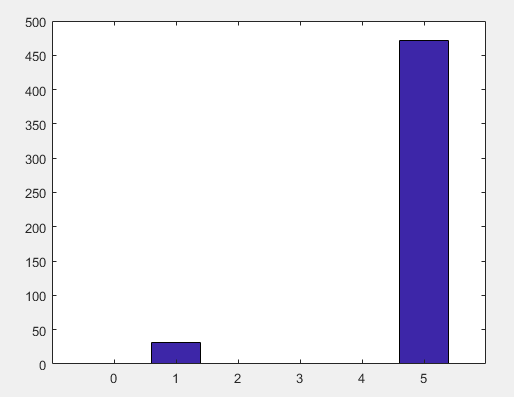结果分析

由于一开始生成的信号，其实是sin(x)跟sin(5x)叠加的信号，因此最后变换后得到的结果符合原结果

代码实现

clear;
format long;
A1 = [100,50];
A2 = [1,5];
N = 1024;
k = 5;

for n = 1:N
x = (2*pi/N)*(n);
y(n) = 10*sin(x) + 30*sin(5*x);
end

w = exp(1i*2*pi/N);
for m = 0:k
c(m+1) = 0.0;

for j = 0:N-1
c(m+1) = c(m+1) + y(j+1)*(w^(m*j));
end
%c(m) = c(m)/N;
result(m+1) = m;
end

%plot(result, c);
bar(result,c);


展开全文• ## 快速傅里叶变换（MATLAB实现）

万次阅读 多人点赞 2018-12-29 15:51:29
一、快速傅里叶介绍 傅立叶原理表明：任何连续测量的时序或信号，都可以表示为不同频率的余弦（或正弦）波信号的无限叠加。FFT是离散傅立叶变换的快速算法，可以将一个信号变换到频域。那其在实际应用中，有哪些...
• 大纲从积分变换谈起积分变换的基本概念积分变换的来源傅里叶级数傅里叶级数的相关基础概念傅里叶级数的基石——三角函数系及其正交性三角函数系三角函数系的正交性傅里叶级数的含义傅里叶系数的导出傅里叶级数收敛...
• 只分析一维度的 ...正变换： fftw_complex *in = fftw_malloc ( sizeof ( fftw_complex ) * n ); fftw_complex *out = fftw_malloc ( sizeof ( fftw_complex ) * n ); plan_forward = fftw_plan_dft_1d ...
• Y = fftn(X) 使用快速傅里叶变换算法返回 N 维数组的多维傅里叶变换。N 维变换等于沿 X 的每个维度计算一维变换。输出 Y 的大小与 X 相同。 Y = fftn(X,sz) 将在进行变换之前根据向量 sz 的元素截断 X 或用尾随零...
• 《用matlab画出余弦函数傅里叶变换图像》由会员分享，可在线阅读，更多相关《用matlab画出余弦函数傅里叶变换图像(3页珍藏版)》请在人人文库网上搜索。1、用matlab画出函数和余弦函数傅里叶变换一、由狄拉克...
• 1、班级：姓名：学号：Matlab函数fft、fft2和fftn分别可以实现一维、二维和N维DFT算法，而函数ifft、ifft2和ifftn则用来计算反DFT，它们是以需要进行反变换的图像作为输入参数，计算得到的输出图像。这些函数的调用...
• 最近做仿真实验，有时需要用傅里叶变换时，老是需要先写写参数再经 过变换，为了解决这个麻烦事，就写个fft变换函数代码，下次直接带入 就方便多了，当然鉴于许多同志当然也包括我对fft这玩意百思不得其解， 不过...
• 式中，y是序列，Y是序列的快速傅里叶变换。y可以是一向量或矩阵，若y为向量，则Y是y的FFT，并且与y具有相同的长度。若y为一矩阵，则Y是对矩阵的每一列向量进行FFT。说明：1. 函数fft返回值的数据结构具有对...
• 原文 定义： ﻿MATLAB帮助文件原文  ...​​1....式中，y是序列，Y是序列的快速傅里叶变换。y可以是一向量或矩阵，若y为向量，则Y是y的FFT，并且与y具有相同的长度。若y为一矩阵，则Y是
• ## 傅里叶变换及其实现（MATLAB）

万次阅读 多人点赞 2018-01-09 17:44:16
对应傅里叶变换，频域连续非周期 2. 周期性连续性信号 对应于傅立叶级数，频域离散非周期 3. 非周期离散信号 对应于DTFT（离散时间傅立叶变换），频域连续周期 4. 周期性离散信号 对应于D
• 即原始k点重排后位置： 即时域信号，x(n)，重排序号perm_num = mod([1:n]*sigma,n)+1，则对应重排后的傅里叶变换，频谱顺序与原信号频谱X，经过重排序号perm_num1 = mod([1:n]*inv_sigma,n)+1，位置始终相差1(数论...
• C语言进行离散傅里叶DFT变换~MATLAB验证C语言进行离散傅里叶DFT变换~MATLAB验证根据离散傅里叶变换的原始公式和自己编写复数计算函数进行离散傅里叶变换对10000个点的加有噪声或干净的正弦波的数据进行离散傅里叶...
• 今天在看图像处理的书时，想绘制一个普通函数的离散傅里叶函数，及领悟函数f(x)*(-1)^x的离散傅里叶变换相当于将原函数的离散傅里叶变换向左或向右平移半个周期。在绘制过程中，想要给图像添加一个坐标轴，发现，...
• matlab 编写快速傅里叶变换函数（ifft）（横向读取数据进行变换） %快速傅里叶变换，不够2的整数幂的个数，末尾自动补齐0
• BB： 最近做脑电信号的时频分析，考虑采用该函数，但在参数设置和输出结果上有些疑惑，参考了众多资料，现对出现的困惑进行一些总结。 如何理解spectrogram()函数的输入输出参数？ Abstract: 我想这篇博文可以帮你弄...信号处理
• C++问题七 C++写Matlab中FFT(快速傅里叶变换)函数 用C++写fft函数可以调用库函数，在这里我调用的库函数是fftw，库下载地址(http://www.fftw.org/install/windows.html)，为：FFTW 3.3.5 Windows DLLs，我下载的是...c++
• 一种积分变换，它来源于函数傅里叶积分表示。积分 (1) 称为ƒ 的傅里叶积分。周期函数在一定条件下可以展成傅里叶级数，而在(－∞，∞)上定义的非周期函数ƒ，显然不能用三角级数来表示。但是J.-B.-J.傅里叶建议把...
• 1.连续时间周期信号的傅里叶级数分析任何一个周期为T的正弦周期信号，只要满足狄利克里条件，就可以展开成傅里叶级数，(至于为什么能展开傅里叶级数和什么是狄利克利条件，这里先不说，我们知道有这样的结论就好)。...
• 根据离散傅里叶变换的原始公式和自己编写复数计算函数进行离散傅里叶变换 对10000个点的加有噪声或干净的正弦波的数据进行离散傅里叶变换，生成10000个点的复数数据序列到文本文件中。 数据格式为实部+虚部，用空格...信号处理 idft
• matlab或R里我们可以直接调用FFT函数实现快速傅里叶变换，然而FFT的输出到底是什么含义，经常让初学者们一头雾水。实际上，它不过是每个采样点(共N个)对应的振幅(可能叫振幅不是很贴切，准确地讲它们的绝对值才是...
• 一、基本概念介绍利用离散傅里叶变换将加噪的调制信号变换到频域，用去除高频的高斯白噪声干扰的方法进行降噪。然后利用希尔伯特变换求得调制信号的解析信号，根据解析式得到调制信号的瞬时参数：瞬时幅度，瞬时频率...
• 目前网络关于分数阶傅里叶变换可靠的代码来源主要有一下几个： （1）来源一： M.A. Kutay. fracF: Fast computation of the fractional Fourier transform, 1996. www.ee.bilkent.edu.tr/~haldun/fracF.m. （2）......

# 傅里叶变换对应的matlab函数matlab 订阅